## Matrix Basix

After all of that Gaussian stuff, our goal is to show you how to use matrices to make things easier in solving systems of equations. But before we do, we need to brush up on all of our matrix basic operations so when you do see the tough stuff, it won't be hard.

This section is all about matrices. We will convert systems of equations to augmented matrices. We'll show you how to add, subtract, and multiply matrices. You'll learn how to find additive inverses, inverses, and transposes of matrices. Lots of stuff that should be a review, but if it's not, you'll get caught up. And we'll even show you a few hints and tricks about using a calculator for matrix operations. Woot.

Okay. Picture a spreadsheet on the computer. Instead, though, it's on paper. You've mastered this section and you haven't even started! Matrices (plural for matrix) are simply spreadsheets on paper.

Matrices look like this: A =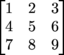Each number in a matrix is called an entry. The columns are vertical and the rows are horizontal. When talking about a specific number in a matrix, we would label it by xrc. If we were talking about the above matrix number 6, we would label it like this: A23 because 6 is in the 2nd row and 3rd column.

Also, the order of the matrix is the row × column. The matrix above is of the order 3 × 3. Kids, you got this. "Ma-trix is for kids."

### Sample Problem

M =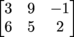What is the order of M, and rewrite this matrix as a system of equations.

The order is 2 × 3 since there are 2 rows and 3 columns. The system of equations will look like this:

3x + 9y = -1
6x + 5y = 2

### Sample Problem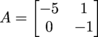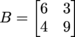Find A + B:

If matrices have the same order (remember the same number of rows and columns), adding and subtracting matrices is a snap. Simply add or subtract the same entry in each matrix.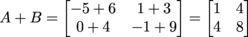### Sample ProblemFind 2BA:

We want to multiply matrix B by 2, which is called scalar multiplication, then subtract matrix A.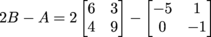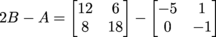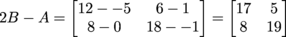### Sample ProblemFind AB:

We want to multiply the matrices together, but this is NOT scalar multiplication. We suggest you put your fingers on the computer screen and follow along as we multiply.

Step 1: Put your left index finger on the A matrix, and your right index finger on the B matrix.

Step 2: Point to -5, then 1, while your right hand is pointing to 6 and then 4.

Step 3: Point again to -5, then 1, while your right hand is pointing to 3 and then 9.

Step 4: Point to 0, then -1, while your right hand is pointing to 6 and then 4.

Step 5: Point again to 0, then -1, while your right hand is pointing to 3 and then 9.

Here's the math: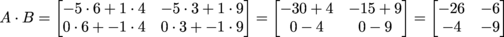### Sample Problem

Find the additive identity of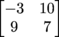Wascally wabbit, "ma-trix" are for kids. Okay, we've already used that joke but since we are talking about wabbits, here's a little trick to pull out of the hat. The additive inverse just means what matrix would you have to add to the matrix to get a matrix full of 0's.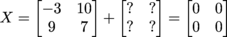Well, that's simple. Our additive identity is: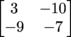### Sample Problem

Find AT (the transpose) of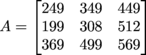The transpose is where you flip the rows and columns. The left column becomes the top row. Or in more technical terms, we are going to reflect all of the numbers of the diagonal drawn from the top left corner to the bottom right corner.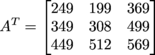The identity matrix is a square matrix (must have the same number of columns and rows) and looks like this:When we start solving equations in the next section, the identity matrix will become very handy. Here is why: if B is the inverse of A, then:

AB = BA = I

I stands for the identity matrix.

Let's try to find the inverse of X also known as X-1.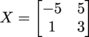Let's think of this problem this way: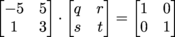It would take a long time to find the inverse matrix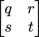because we would have to use matrix multiplication like this: 5q + 5s = 1, etc. We would end up with a system of equations to solve for q, r, s, and t. To save us the hassle, there is a formula to find the inverse of a matrix, just keep in mind it has to be a square matrix.

Here's the formula: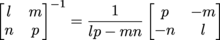You cannot find the inverse of a non-square matrix. Also, you cannot find the inverse of a matrix where the determinant (which is the lp – mn) is 0 in the above equation because you cannot divide by 0 (please don't try or a black hole will form on your computer).

Using the above equation, the inverse will be: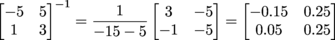It's very easy to find the inverse of a matrix on a calculator. One of the most common calculators to use in high school math is the TI-84 Plus. Here are the instructions for finding the inverse of a matrix on the calculator:

• 2nd MATRIX (which is the X-1 button), arrow twice right to the EDIT, Enter on A

• Type the row and column dimension and press Enter

• Type in the matrix entries

• 2nd QUIT to get back out to the main menu

• 2nd MATRIX, press Enter to have the calculator type A

• Press the X-1 and press Enter

• Attach a chain to your calculator, and wear it around your neck for safe keeping. If anybody asks, tell them you are hooked into the Matrix and cannot speak for yourself.